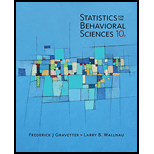# A sample of difference scores from a repeated-measures experiment has a mean of M d = 3 with a standard deviation of s = 4 . a. If n = 4 , is this sample sufficient to reject the null hypothesis using a two-tailed test with α = .05 ? b. Would you reject H 0 if n = 16 ? Again, assume a two-tailed test with α = .05 . c. Explain how the size of the sample influences the likelihood of finding a significant mean difference.### Statistics for The Behavioral Scie...

10th Edition
Frederick J Gravetter + 1 other
Publisher: Cengage Learning
ISBN: 9781305504912

#### Solutions

Chapter
Section### Statistics for The Behavioral Scie...

10th Edition
Frederick J Gravetter + 1 other
Publisher: Cengage Learning
ISBN: 9781305504912
Chapter 11, Problem 19P
Textbook Problem
15 views

## A sample of difference scores from a repeated-measures experiment has a mean of M d = 3 with a standard deviation of s = 4 . a. If n = 4 , is this sample sufficient to reject the null hypothesis using a two-tailed test with α = .05 ? b. Would you reject H 0 if n = 16 ? Again, assume a two-tailed test with α = .05 . c. Explain how the size of the sample influences the likelihood of finding a significant mean difference.

To determine

Do these data indicate a significant mean difference. How the size of the sample influences the likelihood of finding a significant mean difference.

### Explanation of Solution

We are given to use a two-tailed test. That's why alternative hypothesis has sign.

1. The critical value of t values for the critical region are t=±3.182 . So, reject null hypothesis if t<3.182 or t>3.182 .
2. The critical value of t values for the critical region are t=±2.131 . So, reject null hypothesis if t<2.131 or t>2.131 .

Given:

1. M D =3 , n=4 , S D =4 and α=0.05 .
2. M D =3 , n=16 , S D =4 and α=0.05 .1

Formula used:

df=n1 t= M D μ D S D / n

Calculation:

1. When n=4 :

STEP 1: State the hypotheses. The null hypothesis states that there is no significant mean difference. In symbols:

H 0 : μ D =0

The alternative hypothesis states that there is significant mean difference. In symbols:

H a : μ D 0

STEP 2: Locate the critical region. Degree of freedom is:

df=n1 =41 =3 From the t distribution table, for a two-tailed test with α=0.05 for df=3 , the critical value of t values for the critical region are t=±3.182 .

STEP 3: Compute the test statistic. The test statistic is:

t= M D μ D S D / n = 30 4/ 4 =1

### Still sussing out bartleby?

Check out a sample textbook solution.

See a sample solution

#### The Solution to Your Study Problems

Bartleby provides explanations to thousands of textbook problems written by our experts, many with advanced degrees!

Get Started

Find more solutions based on key concepts
Revenue of a Company Williams Commuter Air Service realizes a monthly revenue of 8000x 100x2 (0 x 80) dollar...

Applied Calculus for the Managerial, Life, and Social Sciences: A Brief Approach

8. If find and .

Mathematical Applications for the Management, Life, and Social Sciences

9. List four mortgage loan closing costs. (14-4)

Contemporary Mathematics for Business & Consumers

Sometimes, Always, or Never: The antiderivative of an elementary function is elementary.

Study Guide for Stewart's Single Variable Calculus: Early Transcendentals, 8th

Self Check Evaluate: 10002010-1211-2-21131.

College Algebra (MindTap Course List)

Exponential DecayAn amount A is initially 25. To find next decades amount, we multiply this decades amount by 0...

Functions and Change: A Modeling Approach to College Algebra (MindTap Course List)